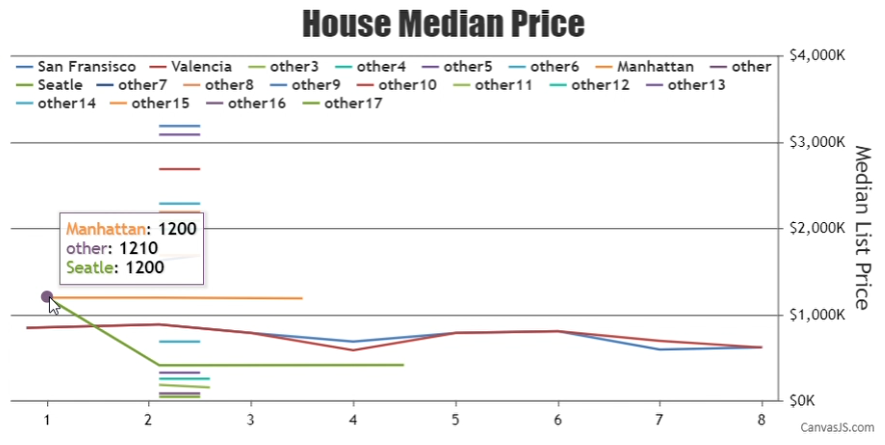Home Forums Chart Support Tooltip multiline chart Reply To: Tooltip multiline chart

#33251

You can show the dataPoints that are close to each other by selecting the dataPoints that fall within a specific range to the mouse pointer position. You can define the range by setting the following variables –

``````var yRange = 100;
var xRange = 10;``````

Then inside the toolTip contentFormatter you can display the dataPoints that fall within the given range as shown below –

``````toolTip: {
contentFormatter: function(e){
var content = "", dataPoint;
toolTipXValue = e.entries.dataPoint.x;
toolTipYValue = e.entries.dataPoint.y;

for (var i = 0; i < e.chart.data.length; i++) {
dataPoint = e.chart.data[i].dataPoints[e.entries.index];
if(dataPoint && (toolTipYValue - yRange) <= dataPoint.y && dataPoint.y <= (toolTipYValue + yRange) && (toolTipXValue - xRange) <= dataPoint.x && dataPoint.x <= (toolTipXValue + xRange)){
content += "<span style='color:" + e.chart.selectedColorSet[i] + "'>" + e.chart.data[i].name + "</span>: " + "<strong>" + dataPoint.y + "</strong>";
content += "<br/>";
}
}
return content;
}
},``````

Please take a look at this JSFiddle for the complete code.___________
Indranil Deo
Team CanvasJS